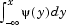# intwave

Integrate wavelet function psi (ψ)

## Syntax

```[INTEG,XVAL] = intwave('wname',PREC) [INTDEC,XVAL,INTREC] = intwave('wname',PREC) [INTEG,XVAL] = intwave('wname',PREC) [INTEG,XVAL] = intwave('wname',PREC,0) [INTEG,XVAL] = intwave('wname') [INTEG,XVAL] = intwave('wname',8) intwave('wname',IN2,IN3), PREC = max(IN2,IN3) intwave('wname',0) intwave('wname',8,IN3) intwave('wname') intwave('wname',8) ```

## Description

`[INTEG,XVAL] = intwave('wname',PREC)` computes the integral, `INTEG`, of the wavelet function ψ (from −∞ to `XVAL` values):for x in `XVAL`.

The function ψ is approximated on the 2PREC points grid `XVAL` where `PREC` is a positive integer. `'wname'` is a character vector containing the name of the wavelet ψ (see `wfilters` for more information).

Output argument `INTEG` is a real or complex vector depending on the wavelet type.

For biorthogonal wavelets,

`[INTDEC,XVAL,INTREC] = intwave('wname',PREC)` computes the integrals, `INTDEC` and `INTREC`, of the wavelet decomposition function ψdec and the wavelet reconstruction function ψrec.

`[INTEG,XVAL] = intwave('wname',PREC)` is equivalent to `[INTEG,XVAL] = intwave('wname',PREC,0)`.

`[INTEG,XVAL] = intwave('wname')` is equivalent to `[INTEG,XVAL] = intwave('wname',8)`.

When used with three arguments ```intwave('wname',IN2,IN3), PREC = max(IN2,IN3)``` and plots are given.

When `IN2` is equal to the special value 0, `intwave('wname',0)` is equivalent to `intwave('wname',8,IN3)`.

`intwave('wname')` is equivalent to `intwave('wname',8)`.

`intwave` is used only for continuous analysis (see `cwt` for more information).

## Examples

```% Set wavelet name. wname = 'db4'; % Plot wavelet function. [phi,psi,xval] = wavefun(wname,7); subplot(211); plot(xval,psi); title('Wavelet'); % Compute and plot wavelet integrals approximations % on a dyadic grid. [integ,xval] = intwave(wname,7); subplot(212); plot(xval,integ); title(['Wavelet integrals over [-Inf x] ' ... 'for each value of xval']); ```## Algorithms

First, the wavelet function is approximated on a grid of 2PREC points using `wavefun`. A piecewise constant interpolation is used to compute the integrals using `cumsum`.

## Version History

Introduced before R2006a